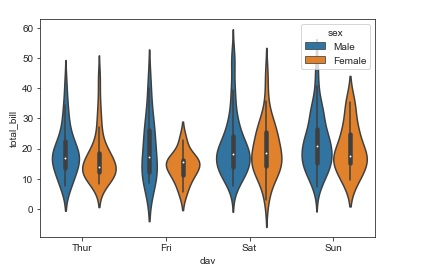# How can every violin in a violin plot be split in Python Seaborn Library?

Seaborn is a library that helps in visualizing data. It comes with customized themes and a high level interface.

Violin plot is a combination of box plot with kernel density estimates (KDE). It is easier to analyse and understand how the data has been distributed. The wide portion of the violin indicates the higher density of data. The narrow portion of the violin indicates the lower density of data.

The inter-quartile range within a boxplot and the higher density portion of data fall within the same region in every category.

Syntax of violinplot function

seaborn.violinplot(x, y, hue, data,…)

Let us see how a split can be made in every violin plot −

## Example

import pandas as pd
import seaborn as sb
from matplotlib import pyplot as plt
sb.violinplot(x = "day", y="total_bill",hue = 'sex', data = my_df)
plt.show()

## Output## Explanation

• The required packages are imported.
• The input data is ‘iris_data’ which is loaded from the scikit learn library.
• This data is stored in a dataframe.